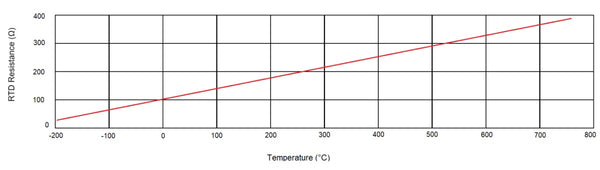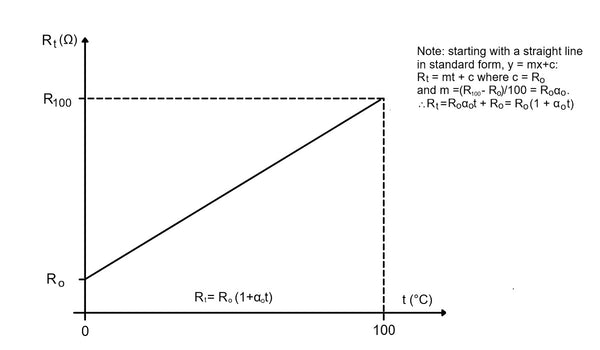# RTD Resistance to Temperature Calculator

Are you looking for a fast way to calculate RTD resistance to temperature? This online RTD conversion calculator determines the optimal temperature value for Pt100, Pt500, and Pt1000 ohms (Ω) platinum resistance thermometers.

Some people can't rely solely on a resistance calculator to determine precise temperature. Do you want to learn how to calculate the temperature from resistance by yourself? As a bonus, we'll tell you the fundamental RTD temperature calculation formulas.

## Online Resistance vs Temperature Calculator

RTD resistance (ohms):

This temperature & RTD calculator supports different types of platinum resistance thermometers (Pt10-Pt1000 ohms to degrees).

To use our calculator:

1. Enter RTD temperature resistance value (the initial resistance value of 100 ohms set to 0°C).

2. Enter the temperature value in °C.

3. Calculate temperature coefficient value (°C) and resistance RTD value (ohms - Ω).

Sound complicated? Let's break down the basics.

## Why Convert Resistance to Temperature?The Resistive Temperature Detector (RTD) is a sensor used for accurate temperature measurement. This device can calculate temperature values precisely with less than a 0.1°C (32.18°F) margin of error.

Many industrial, medical, and commercial processes require specific temperature ranges. That's understandable, given that the temperature value affects energy consumption, chemical, reactions, plants, and health conditions.

You can calculate temperature with different devices, like thermistors and thermocouples. However, RTDs offer the most accurate measurements in any given environment. You can use the reading with RTD (ohms) to degrees calculator to determine the optimal temperature.

The accuracy depends on the combination of base resistance tolerance and temperature resistance tolerance coefficients. The platinum resistance thermometers (Pt) used in RTDs use platinum elements that increase resistance with temperature. In other words, the resistance of the Pt increases along with the sensor's temperature.

The RTD temperature calculation is usually made with 100 ohms resistance at 0° C. The most common types of platinum resistance thermometers are:

• Pt100 (100 ohms resistance at 0°C);

• Pt500 (500 ohms resistance at 0°C);

• Pt1000 (1000 ohms resistance at 0°C).

So, how do you calculate RTD resistance to temperature? Let's find out.

## How to Calculate the Resistance to Temperature?You can relate resistance to temperature with the following RTD calculation formula:

Rt = Rref × [1 + α × (T − Tref)]

In this equation, Rt refers to the RTD Resistance at a given temperature (in ohms), Rref means RTD Resistance at the referenced temperature (in ohms), and α is the temperature-resistance coefficient (in ohms per ohm/degree). The ohms are often shown as Ω in the equation.

The T and Tref stand for temperature and reference temperature. Now, how to calculate the temperature from RTD resistance using this formula? Here's an example.

### RTD Temperature Calculation Formula

For example, let's assume you have a Pt100 RTD sensor. With a 35°C temperature, 0.00392 temp coefficients value, and 0°C reference temp. Your RTD calculation formula will go like this:

RT = 100 x Ω x [ 1 + (0.00392) × (35 − 0) ] = 113.72 ohms

We should note that the temperature is measured in Celsius in every equation and example. You can convert Celsius to Fahrenheit using online calculators. Alternatively, you can use this formula: ºF = ( °C × 9/5 ) + 32

## Conclusion

Now, you understand the basic principles and formulas for RTD temperature calculation. And make sure to check our online RTD calculator to get precise values for RTD resistance and temperature!

If you require high-quality HVAC, controls, or electrical supplies - browse the Blackhawk Supply store today! We offer a wide range of products from trusted manufacturers, so our customers can rest assured that all they need can always be found in one place.

Free Shipping On
Orders over \$999*

Excellent Customer
Satisfaction

Secure Online
Payment

5-star Customer
Service

Quantity
Discounts